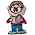## Thursday, August 12, 2010

If:
O plus T = T
O plus S = S
T plus T = F
F plus F = E
E plus O = N

What is N - N?

#### 6 comments:

1.N - N = O

2.Oh. I almost commented saying, "Is this a trick? N-N is zero." Then I realized you were looking for which LETTER would represent N-N. I gotcha. (And Anonymous is right: it's O.)

3.Wouldn't N-N=Z?

O isn't zero in this problem, it's one. The letters are the first letter of the numbers. The problem would read "one plus two = three, one plus six = seven, two plus three = five, four plus four = eight, eight plus one = nine." So, N-N, or nine minus nine, equals zero, which is Z.

4.The answer should be N-N = Z

The key is
One plus Two = Three
One plus Six = Seven
Two plus Two = Four (or Two plus Three = Five)
Four plus Four = Eight
Eight plus One = Nine

And so Nine - Nine = Zero.

5.The answer should be N-N = Z
tht a tricky qsn
The key
One plus Two = Three
One plus Six = Seven
Two plus Two = Four (or Two plus Three = Five)
Four plus Four = Eight
Eight plus One = Nine

And so Nine - Nine = Zero.

6.Ohhhh...now I get it.

Leave your answer or, if you want to post a question of your own, send me an e-mail. Look in the about section to find my e-mail address. If it's new, I'll post it soon.

Please don't leave spam or 'Awesome blog, come visit mine' messages. I'll delete them soon after.

Enter your Email and join hundreds of others who get their Question of the Day sent right to their mailbox

Preview | Powered by FeedBlitz

The Lamplight Manor Puzz 3-DAre you looking for a particular puzzle, riddle, question, etc? Or do you want to find the answer today rather than wait till tomorrow!Web statspuzzles.blogspot.com